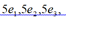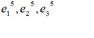## June 12, 2019

Note : We have tried upload as much as we can, all the question and answers might be shuffled - Answers are marked in green. Thanks for Murthy,Abirami who helped us on this quiz.

Questions:

1. In R^3   the four vectors (1,2,5)  (5,9,3) (329,431,731) and (21, -13,58) are
Select one:
A. Linearly dependent
B. Every linear combination is zero
C. Linearly Independent
D. Forms a basis for R^3

2.

Select one:
A. 45
B. 0
C. 10
D. 3

3. The elements along principal diagonal of a Hermitian matrix are all

Select one:
A. either zero or purely imaginary
B. real
C. 0
D. imaginary

4. For the linearly dependent vectors  X=[3,-9,12] and Y=[-4,12,-16]:

Select one:
A. X-2Y=0
B. 4X+3Y=0
C. 4X-3Y=0
D. 3X+4Y=0

5. If A and B are two orthogonal matrices, each of order n then AB and BA are

Select one:
A. Orthogonal
B. asymmetric
C. symmetric
D. Hermitian

6. A set of single non-zero vector is

Select one:
A. Basis
B. None
C. Linearly dependent
D. Linearly Independent

7.Which one of the following is false :

Select one:
A. Matrices is an arrangement while determinant is a value of square matrices.
B. If Rank of A= Rank of B
C. Row equivalent matrices have the same rank.
D. A linearly independent set in v consisting on a maximum possible no of vectors in V is called a basis for V

The correct answer: If Rank of A= Rank of B

8. The Gauss-Elimination method, the augmented matrix reduces to…..................matrix

Select one:
a. Upper triangular
b. Diagonal
c. unit
d. None of these

9. The Characteristics roots of an orthogonal matrix are of ------ modulus

Select one:
A. Unit
B. Two
C. Three
D. Zero

10.Which of the following is not a elementary transformation?

Select one:
b. Squaring all the elements of the matrix
d. Multiplying a row by a non-zero number

The correct answer: Squaring all the elements of the matrix

11.

Select one:
A.

B.C.D.

12. If  rank = number of unknowns  and x = y = z = 0 the equation have only -------- solution

Select one:
A. Non trivial
B. Infinite
C. Unique
D. Trivial

13.

Select one:
A. 3
B. 9
C. 1
D. -3

14.If r is the rank of the matrix [A] of order m x n then r is

Select one:
A. r is less than or equal to minimum of (m, n)
B. r is greater than or equal to 'm'
C. r is greater than 'n'
D. r is less than or equal to 'n'

The correct answer: r is less than or equal to 'n'

15. The standard basis of an inner product space R x R x R (R) forms  an

Select one:
A. Orthonormal set
B. Orthogonal set and Orthonormal set
C. Orthogonal set
D. Neither Orthogonal set nor Orthonormal set

The correct answer: Orthogonal set and Orthonormal set

16. Let A be a matrix having rank ‘r ' & B be the equivalent matrix obtained from A. Then rank of   matrix B is…

Select one:
A. r + 2
B. r -1
C. r
D. r + 1

17. State : which one is false :
Select one:
A. If A and B are Hermitian: then AB-BA is skew Hermitian

B.

C. If A  is a  skew Hermitian : then iA  is Hermitian

D.

18.

Select one:
A. 6,6
B. 6,-12
C. 12,6
D. -6,12.

19. The linear transformation Y = AX is regular if

Select one:
A. I A I#0
B. I A I=1
C. I A I=-1
D. I A I=0

The correct answer: I A I#0

20. A square matrix A of order 3 has 3 linearly independent Eigen vectors then a matrix P can be found such that   P-1AP is a

Select one:
A. Diagonal Matrix
B. Symmetric matrix
C. Singular matrix
D. Unit matrix

1.Huge respect guys... You're doing great...

1.Thank you :)

2.Please update the corrected answers after evaluating the quiz from BITS, so it may helpful fr others.

15) The standard basis of an inner product space R x R x R (R) forms an

Select one:
A. Orthogonal set
B. Orthogonal set and Orthonormal set
C. Neither Orthogonal set nor Orthonormal set
D. Orthonormal set Incorrect
Feedback
The correct answer is: Orthogonal set and Orthonormal set

20)A square matrix A of order 3 has 3 linearly independent Eigen vectors then a matrix P can be found such that P-1AP is a

Select one:
A. Diagonal Matrix
B. Singular matrix
C. Unit matrix
D. Symmetric matrix Incorrect
Feedback
The correct answer is: Diagonal Matrix

14)If r is the rank of the matrix [A] of order m x n then r is

Select one:
A. r is greater than or equal to 'm'
B. r is less than or equal to minimum of (m, n) Incorrect
C. r is less than or equal to 'n'
D. r is greater than 'n'
Feedback
The correct answer is: r is less than or equal to 'n'

5)If A and B are two orthogonal matrices, each of order n then AB and BA are

Select one:
A. Orthogonal Incorrect
B. Hermitian
C. symmetric
D. asymmetric
Feedback

19)The linear transformation Y = AX is regular if

Select one:
A. I A I=-1
B. I A I=1 Incorrect
C. I A I=0
D. I A I#0
Feedback
The correct answer is: I A I#0The linear transformation Y = AX is regular if

Select one:
A. I A I=-1
B. I A I=1 Incorrect
C. I A I=0
D. I A I#0
Feedback
The correct answer is: I A I#0

Thanks for the help

3.